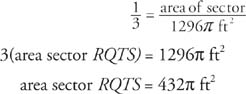## Arc Length and Sectors

Students are often confused by the fact that the arcs of a circle are capable of being measured in more than one way. The best way to avoid that confusion is to remember that arcs possess two properties. They have length as a portion of the circumference, but they also have a measurable curvature, based upon the corresponding central angle.

## Arc length

As mentioned earlier in this section, an arc can be measured either in degrees or in unit length. In Figure 1lis a connected portion of the circumference of the circle.Figure 1 Determining arc length.

The portion is determined by the size of its corresponding central angle. A proportion will be created that compares a portion of the circle to the whole circle first in degree measure and then in unit length.

With the use of this proportion, lcan now be found. In Figure 1, the measure of the central angle = 120°, circumference = 2π r, and r = 6 inches.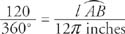Reduce 120°/360° to ⅓.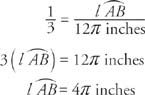Example 1: In Figure 2l= 8π inches. The radius of the circle is 16 inches. Find m ∠ AOB.

Reduce 8π/32π to ¼.Figure 2 Using the arc length and the radius to find the measure of the associated central angle.So, m ∠ AOB = 90°

sector of a circle is a region bounded by two radii and an arc of the circle.

In Figure 3OACB is a sector.is the arc of sector OACB. OADB is also a sector.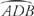is the arc of sector OADB. The area of a sector is a portion of the entire area of the circle. This can be expressed as a proportion.Figure 3 A sector of a circle.

Example 2: In Figure 4, find the area of sector OACB.Figure 4 Finding the area of a sector of a circle.

Example 3: In Figure 5, find the area of sector RQTS.Figure 5 Finding the area of a sector of a circle.

The radius of this circle is 36 ft, so the area of the circle is π(36)2 or 1296π ft2. Therefore,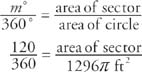Reduce 120360 to ⅓.